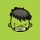Home
IT Knowledge
Inspiration
Languages
EN

# Node.js - save number to file

0 points
Created by:Adnaan-Robin
274

In this article, we would like to show you how to write line to the file in Node.js.

Quick solution:

``````const fs = require('fs');

const number = 123;
const data = number.toString();  // convert the number to string, so you can write it to file

fs.writeFile('./example.txt', data, (error) => {
if (error) {
console.error(error);
return;
}
});``````

Note:

This solution replaces the `example.txt` file contents if it already exists.

In this section, we present how to modify the quick solution from previous section by specifying an additional argument (flag) in `fs.writeFile()`.

FlagDescription
`r+`
• open the file for reading and writing
`a`
• open the file for writing
• positioning the stream at the end of the file
• the file will be created if it doesn't exist
`a+`
• open the file for reading and writing
• positioning the stream at the end of the file
• the file will be created if it doesn't exist
`w+`
• open the file for reading and writing
• positioning the stream at the beginning of the file
• the file will be created if it doesn't exist

Practical example:

``````const fs = require('fs');

const number = 123;
const data = number.toString();

fs.writeFile('./example.txt', data, { flag: 'a+' }, (error) => {
if (error) {
console.error(error);
return;
}
});``````

Note:

Now when you run the program, the `data` string will be added at the end of `example.txt` file (but not in the separate line).

## Alternative solution

In this example, we use `fs.writeFileSync()` as an alternative solution to `fs.writeFile()`. The API also replaces the contents of the file if it does already exist.

``````const fs = require('fs');

const number = 123;
const data = number.toString();

try {
const result = fs.writeFileSync('./example.txt', data); // fs.writeFileSync(path, string);
} catch (error) {
console.error(error);
}``````